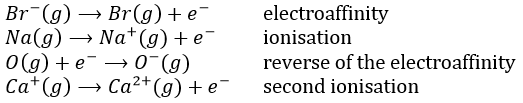# Chapter 1d: Periodic table of elements

The table of Mendeleev is also called the periodic table of the elements. More than a simple list of the existing atoms. Mendeleev sorted the elements with regard to their oxidation/reduction by O/H

R20  R0                                                                                R302  RH4 RH3 RH2 RH   R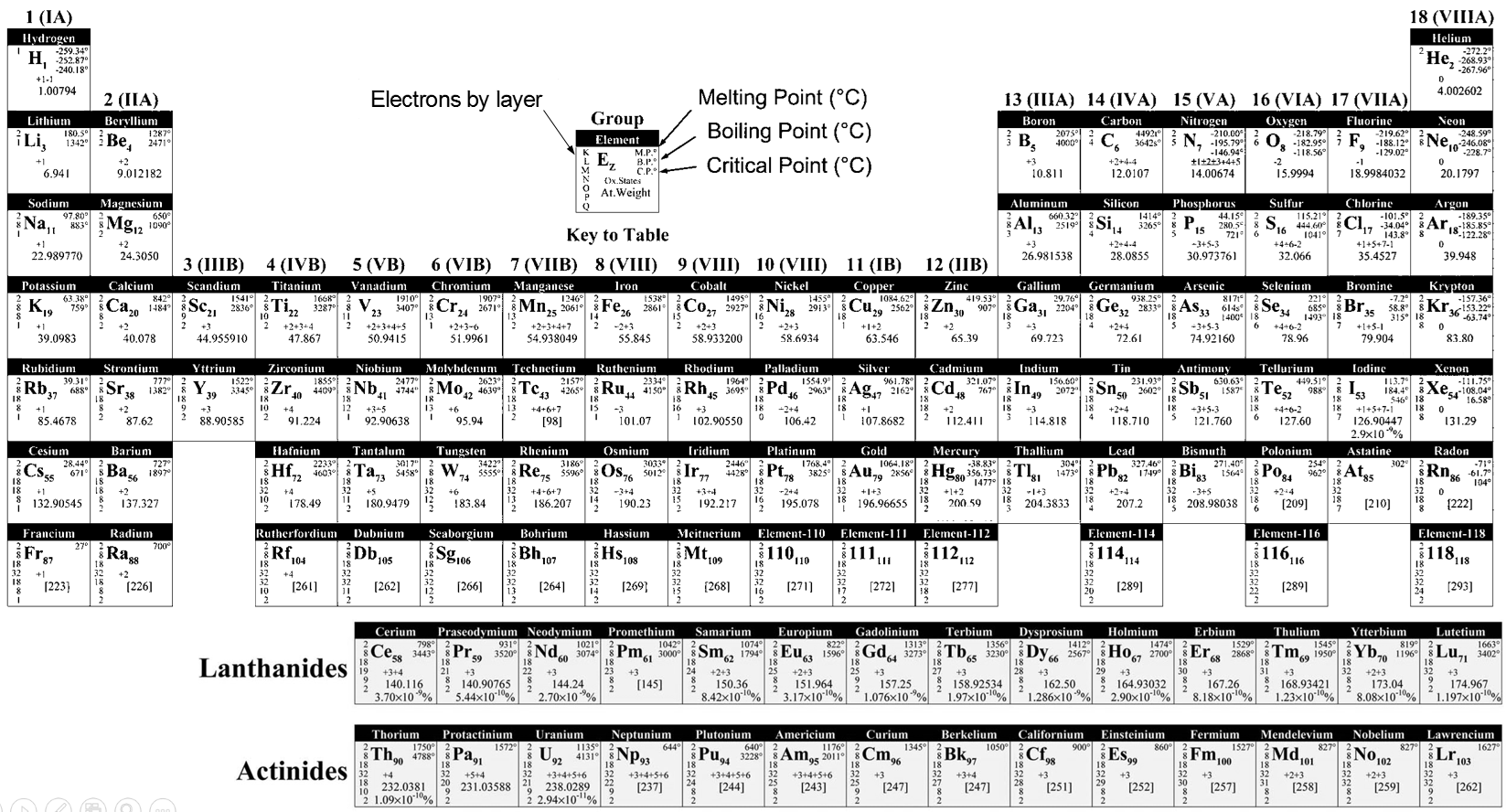Where R is the element. The atoms of same oxidation were sorted by weight to obtain the different lines. Some holes were present in the table but it was assumed that the missing elements were not yet discovered. Scientists have been able to fulfil the periodic table by the artificial synthesis of those elements. In the table showed above, the elements from 110 to 118 are theoretical.

It may not look like, but the determination of this table was a huge improvement for the chemists. The table contains a gigantic number of information for each atom, but also shows some periodic trends.

One case

There is a large number of different models for the periodic tables. Some of them only show the symbol, name, atomic number (Z) and weight of each element but many other information can be displayed for each element. An example follows for the Aluminium.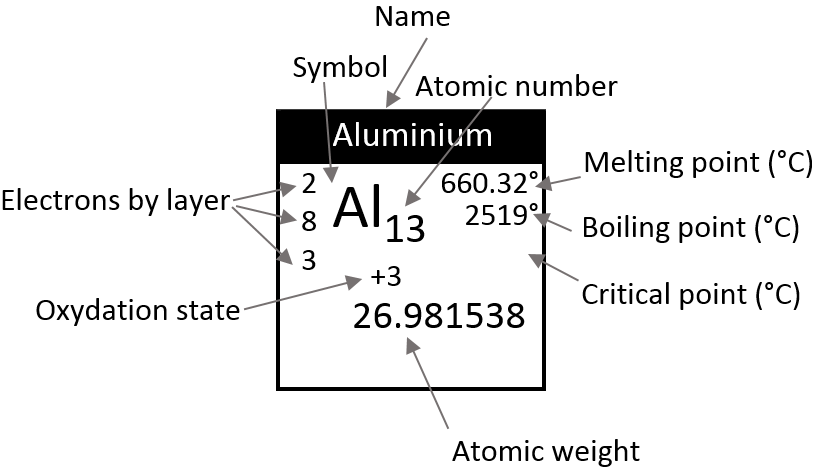Its symbol is Al, its atomic number is 13 and it has a weight of 26.981538 units of atomic mass (which is equivalent to the molar mass, in g/mol).  The weight of an element is the average of the mass of its isotopes, taking in account their proportions. The weight is the property that we seek most of the times on a Mendeleev table. The number of electrons by layer can be determined from Z but is often indicated on the periodic table. Aluminium Z=13, meaning that the layer n=1 is complete with 2 electrons, the layer n=2 is complete with 8 electrons (2 in 2s2 and 6 in 2p6) and that 3 electrons are to be distributed in the n=3 layer. The 2 first electrons are in 3s2 and the last electron is in a 2p orbital. From that, we can determine that Al is on the third line of the table (n=3), in the third column (3 electrons are in the last layer).

Aluminium has a unique oxidation state (OS) of +3 (to be seen in a next section). Elements after the third column can have several OS depending on how they use their electrons. Finally, some temperature are indicated to let us know in which state, solid, liquid or gaseous, the element is found at a given temperature.

Lines

Each line corresponds to a value of n. Because a layer of electrons is added for each line, the radius of the elements increases when we are descending in the periodic table. The number of elements in a line is not identical from line to line, because the orbitals p and d are not present in the first lines. One can also see two particular lines at the bottom of the table, the lanthanides and the actinides, corresponding at the elements possessing an outer nf orbital.

Columns

The elements on a same column have the same number of electrons of valence. Each column is called a family and has a particular name. The three first columns and the 5 last are denoted Ia, IIa, IIIa,…,VIIIa and contain the main elements. In between are the transition metals, noted Ib, IIb,…

Ia (except H): alkali metals: they are all shiny (but tarnish in the contact of air), soft, highly reactive metals (in sctp, standart conditions of temperature and pressure) and readily lose their outermost electron to form a cation. They form strong bases when bound to OH (NaOH,KOH).

IIa: alkaline earth metals: they are all shiny and reactive (in sctp). They readily lose their outermost electrons to form a cation of charge 2+.

IIIa: icosagens or triels: the column of Boron and Aluminium. They have 3 electrons in their outermost layer. Aluminium is one of the rare metals to have a low density.

IVa: crystallogens or tetrels: the group of the carbon and of the silicon. They have 4 valence electrons. Carbon is the essential component of living bodies (~23% of a human) but it is also a constituent of the earth through carbonates, and of the atmosphere through CO2. It is very resistant to heat. Silicon is a major constituent of the earth, second in abundance there. Si and C are in the same column but are surprisingly different. One of their common properties is that they can make long chains (C can make chains much longer than Si though). C can form pi liaisons while Si cannot because its radius is too large (no possible superimposition of the orbitals). CO2 is a gas while SiO2 is a solid (quartz) that is the base of the glass materials.

Va: pnictogens: the group of the nitrogen and of the phosphorus. 5 valence electrons, with two paired. They form stable covalent liaisons and can form double and triple liaisons. This ability to form persistent liaisons is the source of the toxicity of some elements of this group (arsenic, antimony). On the other hand, N2 is an inert gas which represents 78% of the air.

VIa: chalcogens: The group of the oxygen and of the sulphur. Oxygen has very different properties than the rest of this group: they are soft and do not conduct heat well. Oxygen makes up 21% of the atmosphere by weight, 46% of the earth’s crust by weight and 65% of the human body. Oxygen also occurs in many minerals, being found in all oxide minerals and hydroxide minerals, and in numerous other minerals. Ozone is spontaneously formed in the high atmosphere where it catches UV rays from the sun.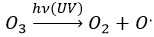The emitted radical will react immediately with an adjacent molecule. The ozone is a better oxidant than O2 because a pi liaison is delocalised. As a result, ozone is often used to kill bacteria’s without waste.

VIIa: Halogens: All the halogens form acids when bound to a hydrogen and are generally toxic. They also from salts when bound to alkali’s.

VIIIa: Noble gas/Rare gas: those element are inert: they do not react with any other element. Helium is the most common element in the universe (~24% of its mass). Because of their lack of reactivity, there are used in lighting (also true for nitrogen)

Metals, metalloids, nonmetals

The periodic table can be divided in three broad sections: metals, metalloids, nonmetals. These three categories are well regrouped in the table (except H, as always). Most of the elements are metals. The nonmetals are on the top right corner of the table (+H) and metalloids are only the elements on a diagonal from Boron to Polonium.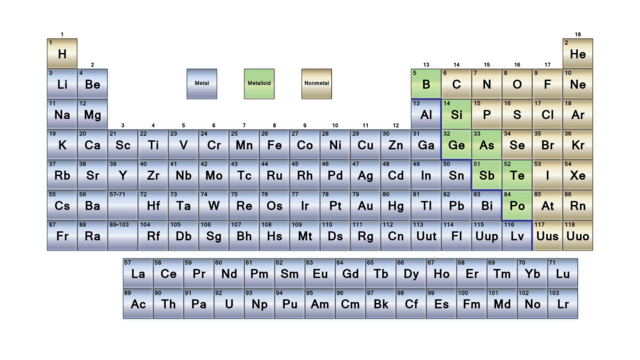Metals

Metals have good thermal and electrical conductivity. As a broad category, they have common properties but exceptions exist for several elements for one or more properties.

They have in general a low ionisation energy, a low electronegativity and give or share their electrons when bonding. The bonding of two or more metals forms an alloy. Most of them can form oxides and are naturally found in this state.

In general, they are soft and malleable solids of high density but some are liquids in sctp (Hg for example). Most of them are silvery coloured.

Nonmetals

Most of nonmetals are low density gases in sctp. When bonding, they share or accept electrons and do not form basic oxides (but well acidic oxides as HClO, H2SO4, etc). In opposition to metals, they do not conduct electricity or heat well, and have a high ionisation energy and electronegativity. Usually, they are not naturally found in a combined state.

Metalloids

They are the minority of the elements. They look like metallic solids and can form oxides (acidic, basic or amphoteric). Most are semiconductors, and moderate thermal conductors, and have structures that are more open than those of most metals.

Periodic properties

Several kinds of radius can be determined for a single element.

Crystalline radius: a crystal is a solid in which the atoms are spatially arranged. Monocrystals are obtained from the aggregation of atoms on one unique atom. They have the advantage of having less defaults in their structure than crystals arranged around several sources. Crystals are not necessarily a structure made of a single element. It is for example usual to make crystals from proteins to determine their spatial structure.

The method to obtain crystals is as follows: the element of the crystal is first dissolved in a solvent. The dissolution can be helped by an increase of the temperature. Once the dissolution is complete, the goal is to decrease very slowly the affinity of the element with the solvent. It can be done through the evaporation of the solvent (another solvent of lower affinity replaces the evaporated solvent) or if the solution was heated, through a slow decrease of the temperature. The process has to be slow to avoid the formation of several centres of aggregation.

The structure of the crystals and the distance between atoms inside a crystal depends on its constitutive elements and can be determined by RX diffraction.

In this method, a RX beam hits the surface of a crystal with a given angle θ.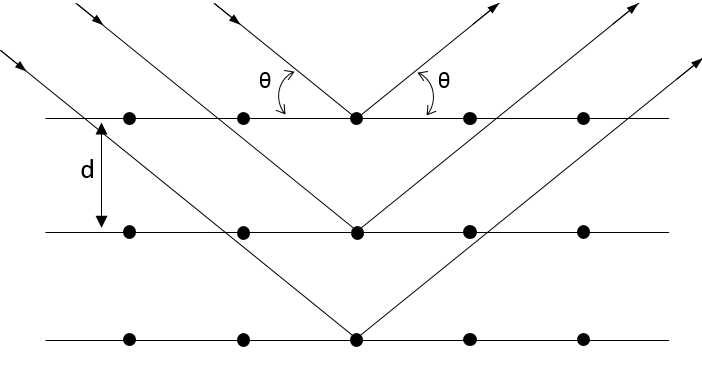The interaction of this beam with the atoms of the first layers of the crystal. This interaction gives place to diffraction only if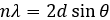Where d is the distance between layers in the crystal, θ the angle of incidence and λ the wavelength of the beam. This equation is the equation of Bragg. If this condition is not fulfilled, the interaction between the atoms and the beam are destructive (i.e. they are cancelling each other) and no diffraction occurs. The radius is the half of the distance between atoms in the crystal.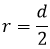Calculated radius: we consider here that the radius for which the probability of presence of the electron is the largest is the radius of the atom.

Van der Waals radiusThe van der Waals radius is obtained by the addition of the repulsive force and the attractive force between two atoms. These two forces depend on the distance separating the atoms but not the same way. The attraction is proportional to the radius power -7 while the repulsion on the radius power -13. As a result, there is a favoured distance between the atoms when they bind. It is the attraction pit. Reducing this distance increases drastically the potential energy. σ is the distance of smallest approach and is the crossing between the curve and Epot=0.  Increasing the distance also increases the potential energy towards 0.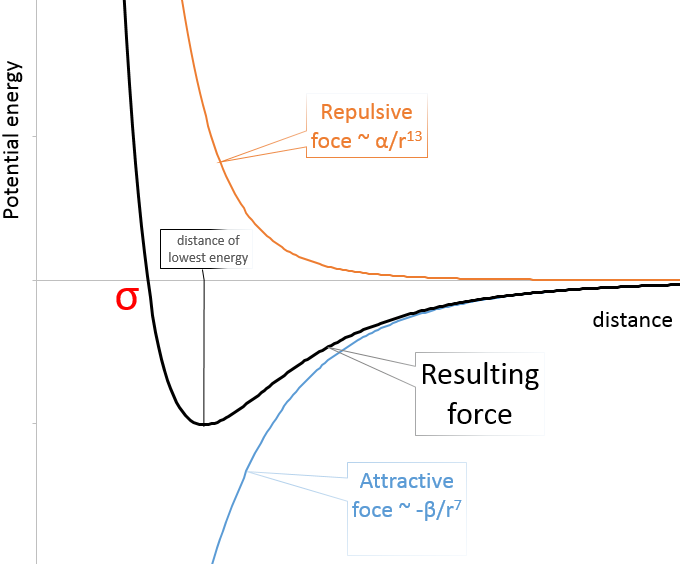The radius of the atom can be determined from σ.

Covalent radius: for diatomic molecules, the radius of the atom is considered as the half of the liaison’s length.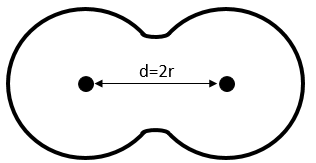Empirical radius: it is obtained from the volume of the atomic gas. In a given volume of gas, there is a given number of atoms. Considering spherical atoms, the volume of one atom is V=4/3π r3. Therefore the radius is determined.

Those different radius are not equals and in some case they do not exist. For example, there is no covalent radius for He.

However, each of them follows the same logical repartition in the periodic table: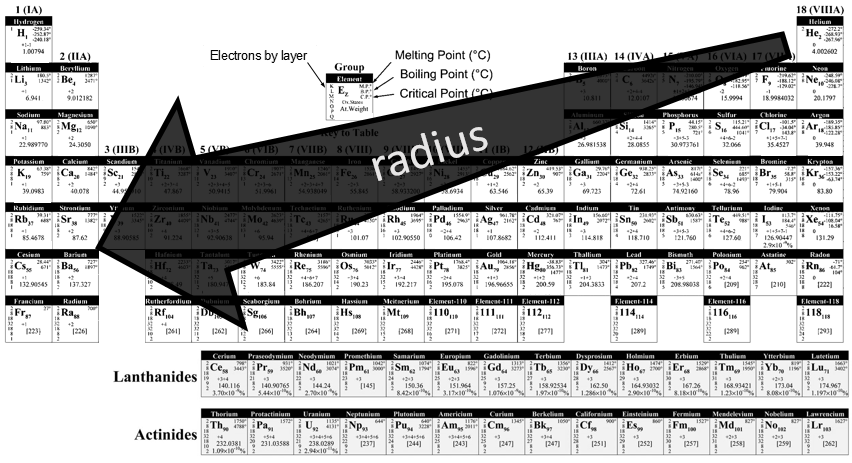As we have seen in the previous section, a layer of electrons is added at each new line of the periodic table. It is thus logical that the radius of the elements increases in a single column. Moving across a line, the number of protons in the nucleus increases and the outer electrons are thus more and more attracted by the nucleus. From left to right, the radius decreases. As a result, Li and Mg have a similar radius, as do Na and Ca.

Ionisation energy

The ionisation energy (IE) is the energy required to remove one electron from an atom of a gas.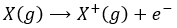This energy is positive (atoms in gas don’t lose their electrons spontaneously) and depends on the energy of the electron: an electron close to the nucleus is hard to remove. It is also way more difficult to remove a second electron from an atom which had already lose one. For example, the aluminium has the following ionisation energies: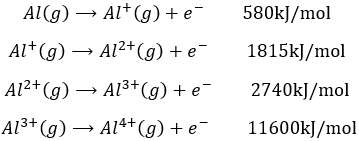The first electron is relatively easy to remove: it is the single electron on the orbital 3p of the aluminium. The next electron is way more difficult to remove: it is an electron on a complete orbital. The third energy of ionisation is small for a third ionisation: we obtain the electronic structure of the noble gas Neon: the octet is complete and this structure is stabilized. This stabilisation is visible in the next energy of ionisation: a gigantic energy is required to remove one additional electron.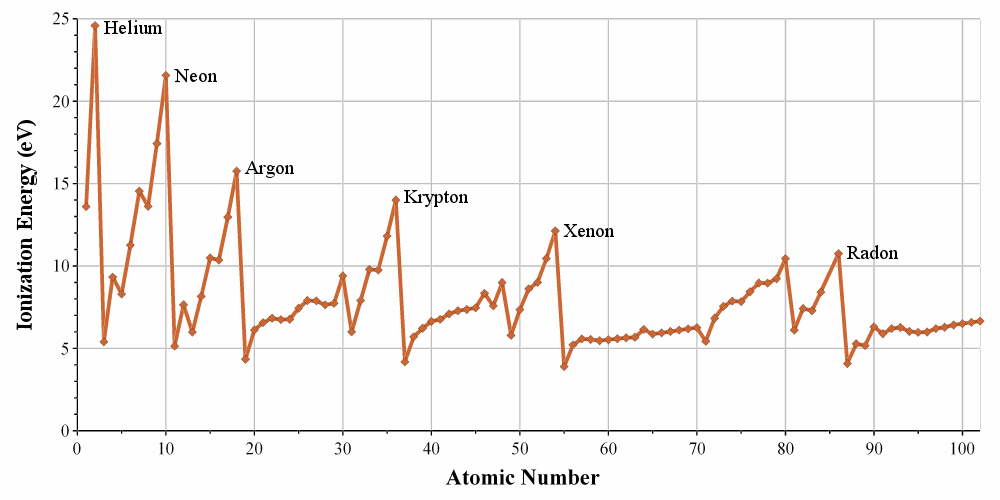The energies of ionisation are periodic on the Mendeleev table. It requires a massive energy to remove an electron from a noble gas but it is easier to go towards its electronic structure. In one period/line, one can see that the trend of the IE is not linear. It is more difficult than average to remove an electron from a complete orbital

It is easier to remove one paired electron (less repulsion)

It is easier to empty one orbital: the last electron is shielded by the electrons of inner orbitals.

For the same last reason, the IE decreases when moving downwards in the periodic table: the shielding effect is bigger and bigger with the number of layers of electrons.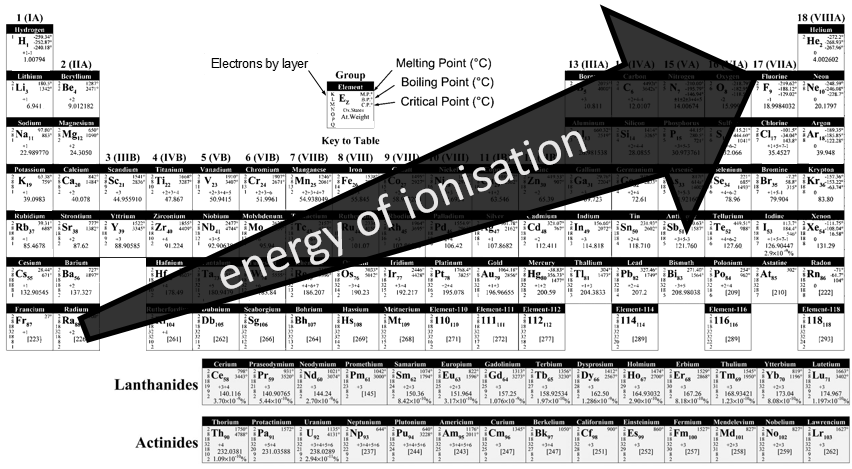Electroaffinity

The electroaffinity is just the opposite of the ionisation: it is the energy required by a gaseous atom to accept an additional electron and follow the opposite evolution than the IE.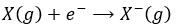Note that noble gases don’t have any electroaffinity because they are inert.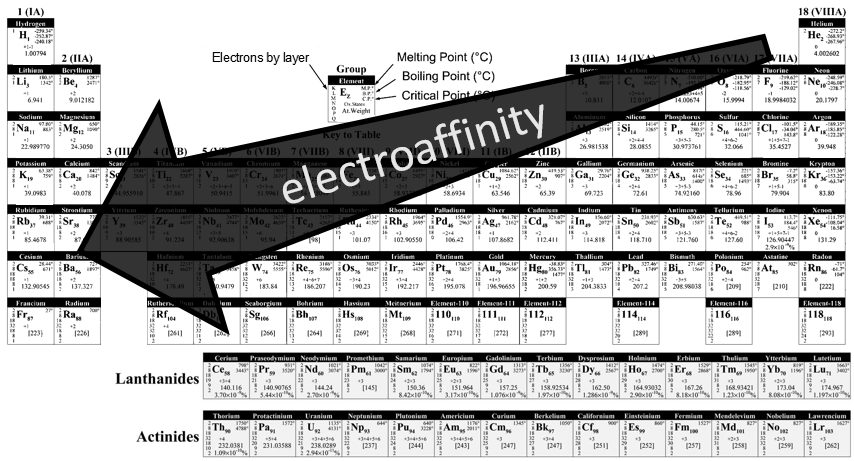Electronegativity

The electronegativity is an important notion in chemistry: it is the ability of an atom to attract the electrons of a liaison it shares. The symbol for the electronegativity is χ.

Pauling determined that, by convention the fluor, being the atom of largest electronegativity, has χ =4. The other elements have an electronegativity given by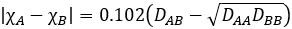Where DXY is the energy of dissociation between X and Y, i.e. the required energy to break a liaison between X and Y.

The fluor being in the top right corner of the table, it is not difficult to understand the trend for the electronegativity.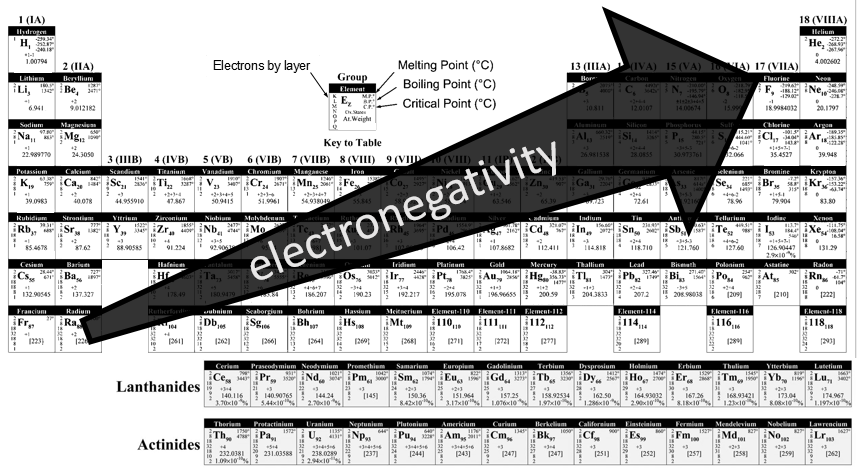Exercises

1. What is the symbol, atomic number and weight of the following species?

Chlorine, silver, sodium, carbon, argon, neon, cerium, magnesium, oxygen, iron, tin, antimony

1. Name the following species:

O2, NaCl, 10n, HNO3, SnBr4, 21H, P2O5, H2S, HClO2, CO2, HClO3, HNO2, HClO4.

1. What is the molar weight of the above cited species?
1. What is the formula of

Carbon monoxide

phosphorous acid

sodium bromide

dinitrogen trioxide

hydrogen peroxide

1. How much perchlorate of calcium do we have to weight if we want 50 moles of it?
2. How many molecules of AgI is there is 20g?
3. Which element has a higher electronegativity?
1. F or I
2. Na or Cl
3. Mg or Ar
4. Which element has a higher energy of ionisation?
1. I or Br
2. C or F
3. P or Na
5. Which element has a larger radius?
1. Al or S
2. Li or O
3. Sn or Rb
6. Chlorine has two stable isotopes: 35Cl and 37 Their masses are 34.96885 and 36.96590. What are is the proportion of both of the isotopes knowing that the atomic mass of the chlorine is 35.4527?
7. The mass of 0.1726mol of an acid HXO4 is 25g. Determine the element behind X.
8. How many valence electron in halogens? In earth-alkalines?
9. What are the names of those reactions?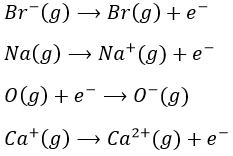1. What is the symbol, atomic number and weight of the following species?

Chlorine, silver, sodium, carbon, argon, neon, cerium, magnesium, oxygen, iron, tin, antimony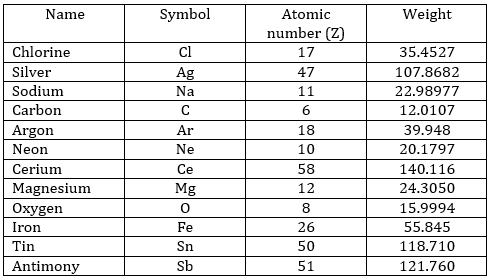1. Name the following species:

O2: oxygen

NaCl: Sodium chloride

10n: neutron

HNO3: nitric acid

SnBr4: tin (IV) bromide

21H: deuterium

P2O5: phosphorus pentoxide

H2S: hydrogen sulphide

HClO2: chlorous acid

CO2: carbon dioxide

HClO3: chloric acid

HNO2,: nitrous acid

HClO4: perchloric acid

1. What is the molar weight of the above cited species?

O2: 32g/mol

NaCl: 58.44g/mol

10n: 1g/mol

HNO3: 63.01g/mol

SnBr4: 438.33g/mol

21H: 2.01g/mol

P2O5: 141.95 g/mol

H2S: 34.08 g/mol

HClO2: 68.46 g/mol

CO2: 44.01 g/mol

HClO3: 84.46 g/mol

HNO2,: 17.18 g/mol

HClO4: 100.46 g/mol

1. What is the formula of

Carbon monoxide: CO

phosphorous acid: H3PO3

sodium bromide: NaBr

dinitrogen trioxide: N2O3

hydrogen peroxide: H2O2

1. How much perchlorate of calcium do we weight if we want 50 moles of it?Formula of the perchlorate of calcium: Ca(ClO4)2Its molar mass is: M=40.078g/mol+ 2x(35.4527g/mol + 4×15.9994g/mol) =238.9786g/molThus, 50mol of it weight 11.948kg.
2. How many molecules of AgI is there is 20g?1 mol of AgI weights 234.77267g. There are thus 0.085mol of AgI in 20g. The question is how many molecules are in 20g of AgI. In one mole, there are NA (the Avogadro number=6.022 1023) molecules. That makes 5.13 1022 molecules.
3. Which element has a higher electronegativity?
1. F or I
2. Na or Cl
3. Mg or Ar (Ar is a noble gas, so it does not accept any additional electron)
4. Which element has a higher energy of ionisation?
1. I or Br
2. C or F
3. P or Na
5. Which element has a larger radius?
1. Al or N
2. Li or O
3. Sn or Rb
6. Chlorine has two stable isotopes: 35Cl and 37 Their masses are 34.96885 and 36.96590. What are is the proportion of both of the isotopes knowing that the atomic mass of the chlorine is 35.4527?

To solve this, we have to consider 2 things:

• The atomic mass of an element is the average of the masses of its stable isotopes. So 35.4527=x 34.96885 + y 36.96590 where x and y are the proportions of each isotope.
• The sum of the proportions of the isotopes is 100%: 1 (100%)= x + y.

We have thus two equations with 2 unknowns. That can easily be solved: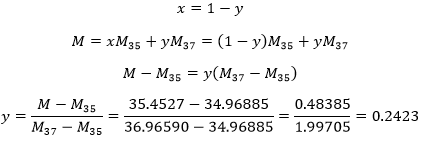The proportions are thus 24.23% of 37Cl and 75.77% of 35Cl.

11.We determine first the molar weight of the acid: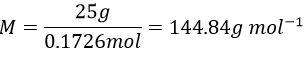The mass of X is the molar weight of the acid minus the known atoms: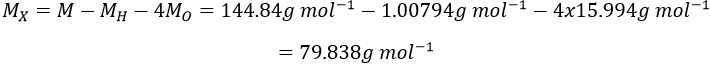X is thus the bromine Br.

12. 7 in halogens, 2 in earth-alkalines

13.